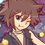# Combinatorics (Thailand Math POSN 1st elimination round 2014)

Write a full solution.

1.1) Find the number of ways that the word GIGGLING can be arranged such that the words can't start with vowels.

1.2) There're 50 numbers from 1 to 50. How many different ways can it be arranged in straight line such that odd numbers are arranged in increasing order and 2,4,6,8 must be next to each other (can be any order).

2.) (I feel like there's a problem in this question) Let $L(n,r)$ be the number of ways of setting $r$ people sit on $n$ identical round tables such that each tables have at least $k$ people. Prove that

$L(n,r) = (r-1)L(n,r-1) + \displaystyle \frac{(r-1)!}{(r-k)!}L(n-1,r-k)$

for all natural numbers $r,n,k$ and $nk \leq r$

3.) Let $n$ be natural numbers, prove these by combinatorial proof.

3.1) $\dbinom{2(n+1)}{n+1} = \dbinom{2n}{n+1} + 2\dbinom{2n}{n} + \dbinom{2n}{n-1}$

3.2) $\displaystyle \frac{\left((n+1)!(n^{2}+5n+7)\right)!}{((n+1)!)^{n!}\left((n+3)!\right)!}$ is an integer.

4.) There're $20$ different gifts, give them to $4$ of the $8$ students (not necessary every gifts are given). If each $4$ students get more than $1$ gifts, find the number of ways to do that.

5.) There're $n$ people in a group (Brilli is one of them). Choose at least $2$ people and have them stand on a straight line. The rest of people, except Brilli, are sitting on a circular table. Find the number of ways to do that.

Check out all my notes and stuffs for more problems!

Thailand Math POSN 2013

Thailand Math POSN 2014Note by Samuraiwarm Tsunayoshi
5 years, 9 months ago

This discussion board is a place to discuss our Daily Challenges and the math and science related to those challenges. Explanations are more than just a solution — they should explain the steps and thinking strategies that you used to obtain the solution. Comments should further the discussion of math and science.

When posting on Brilliant:

• Use the emojis to react to an explanation, whether you're congratulating a job well done , or just really confused .
• Ask specific questions about the challenge or the steps in somebody's explanation. Well-posed questions can add a lot to the discussion, but posting "I don't understand!" doesn't help anyone.
• Try to contribute something new to the discussion, whether it is an extension, generalization or other idea related to the challenge.

MarkdownAppears as
*italics* or _italics_ italics
**bold** or __bold__ bold
- bulleted- list
• bulleted
• list
1. numbered2. list
1. numbered
2. list
Note: you must add a full line of space before and after lists for them to show up correctly
paragraph 1paragraph 2

paragraph 1

paragraph 2

[example link](https://brilliant.org)example link
> This is a quote
This is a quote
    # I indented these lines
# 4 spaces, and now they show
# up as a code block.

print "hello world"
# I indented these lines
# 4 spaces, and now they show
# up as a code block.

print "hello world"
MathAppears as
Remember to wrap math in $$ ... $$ or $ ... $ to ensure proper formatting.
2 \times 3 $2 \times 3$
2^{34} $2^{34}$
a_{i-1} $a_{i-1}$
\frac{2}{3} $\frac{2}{3}$
\sqrt{2} $\sqrt{2}$
\sum_{i=1}^3 $\sum_{i=1}^3$
\sin \theta $\sin \theta$
\boxed{123} $\boxed{123}$

Sort by:

3.1) Consider $2n$ footballers, a cricketer "A" and a tennis player "B". We have to select $n+1$ of them.

DIRECT METHOD :- Total number of ways is ${2n + 2 \choose n+1}$.

INDIRECT METHOD :- There are four cases, (a) Both A and B are selected (b)Only A is selected (c) Only B is selected (d) Neither A nor B is selected.

Number of ways in case (a) = ${2n \choose n-1}$.Since A and B are chosen, we have to select the other $n-1$ players from the footballers.

Number of ways in case (b) = ${2n \choose n}$..Since only A is chosen, we have to select the other $n$ players from the footballers.

Number of ways in case (c) = ${2n \choose n}$.Since only B is chosen, we have to select the other $n$ players from the footballers.

Number of ways in case (d) = ${2n \choose n+1}$.Since neither A nor B is chosen, we have to select the other $n+1$ players from the footballers.

Since both the methods must give the same answer, we can conclude that ${2n+2 \choose n+1} = {2n \choose n+1} + {2n \choose n} + {2n \choose n} + {2n \choose n-1}$

- 5 years, 9 months ago

Nice! You can also use Pascal's identity easily.

- 5 years, 9 months ago